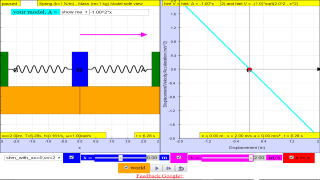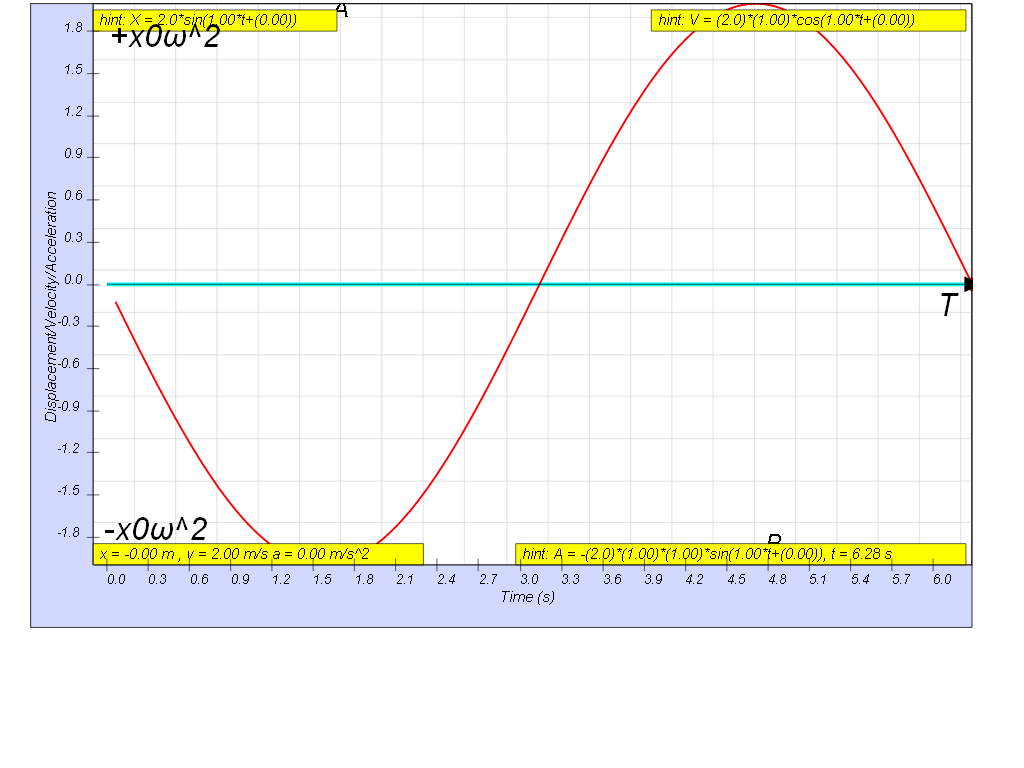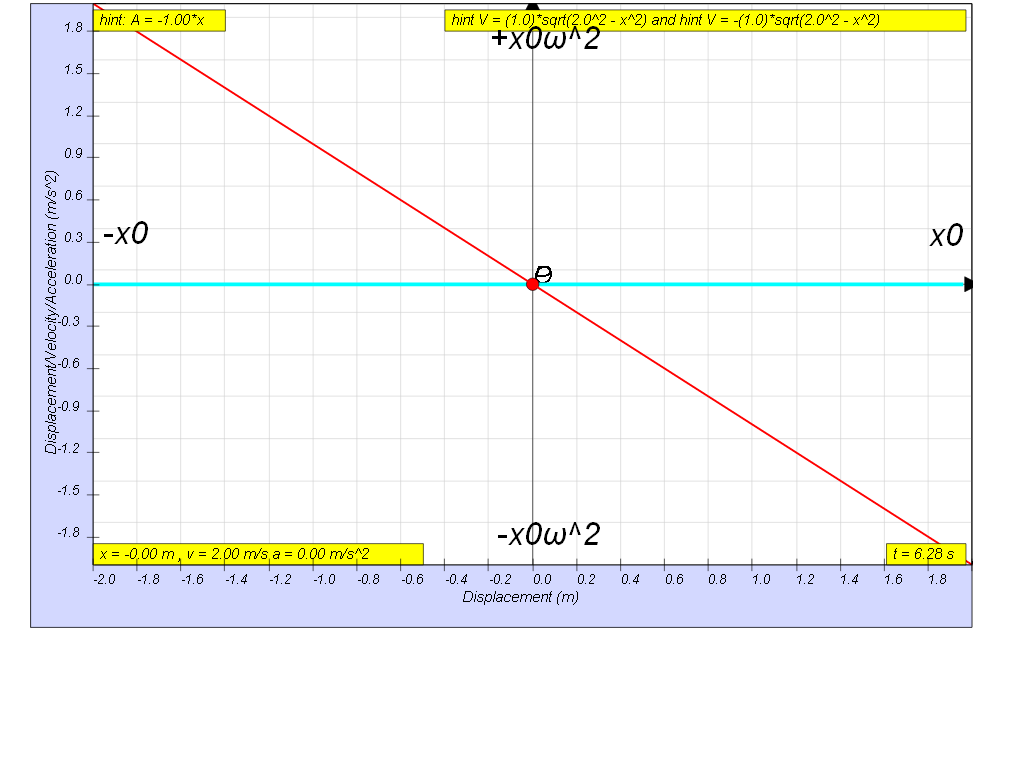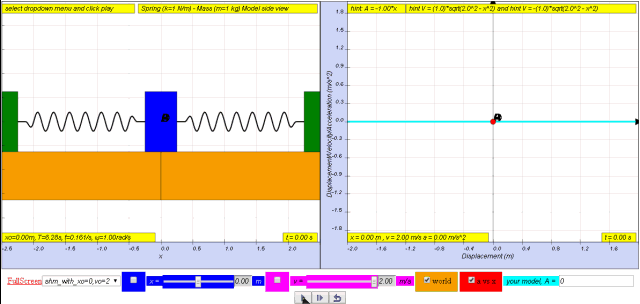SHM10

### 1.2.4 Acceleration

From v = vo cos ω t = x0 ω cos ω t

where xo is the maximum displacement

differentiating we get

$a=\frac{dv}{dt}=-{\omega }^{2}\left({x}_{0}sin\omega t\right)=-{\omega }^{2}x$Variation with time of acceleration

## In terms of x:

Therefore,  a = - xo ω2 sin ω t
= - ω2 (xo sin ω t)
which is the defining equation for S.H.M. !
a     = - ω2 xVariation with displacement of acceleration

since

a = – a0sin ω t

where ao is the maximum acceleration
where by a0 = ω2 (xo)### Translations

Code Language Translator Run### Software Requirements

SoftwareRequirements

 Android iOS Windows MacOS with best with Chrome Chrome Chrome Chrome support full-screen? Yes. Chrome/Opera No. Firefox/ Samsung Internet Not yet Yes Yes cannot work on some mobile browser that don't understand JavaScript such as..... cannot work on Internet Explorer 9 and below

### CreditsThis email address is being protected from spambots. You need JavaScript enabled to view it.

### end faq

http://iwant2study.org/lookangejss/02_newtonianmechanics_8oscillations/ejss_model_SHM10/SHM10_Simulation.xhtml